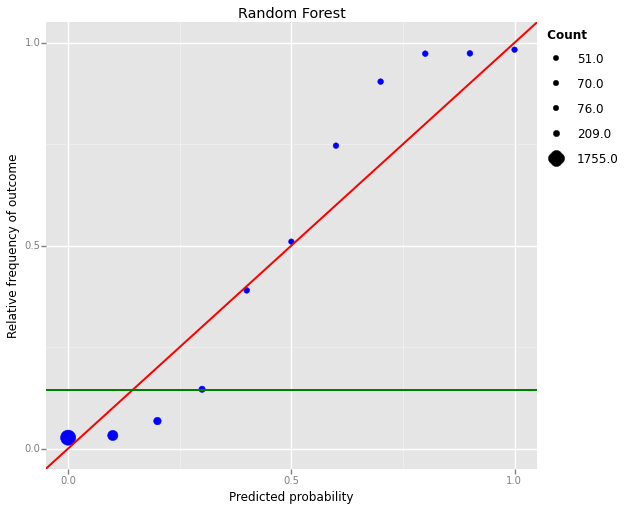Machine Learning: how do we usually select the best combination of parameters to obtain well-calibrated probabilities instead of classification task?

I have always dealt with Machine Learning problems involving classification tasks. I now have the following problem: obtain the purchasing probabilities of users given a certain dataset.

Reading the literature it seems to me that for obtaining well-calibrated probabilities one first has to train the model as a classification problem (with a binary score as precision, recall, f1 etc) and then calibrate the probabilities with isotonic regression or platt scaling. By well-calibrated probabilities I mean resembling the diagonal (perfect prediction) in a reliability plot (predicted probabilities by model vs the relative frequency of positive outcomes).

The following Figure is an example of a NOT well-calibrated problem using Random Forest (red->diagonal, green-> average fraction of purchasing events):To tackle this problem is it enough to do the GridSearchCV (parameter search) with log-loss as a performance metric?.

Another performance metric could be calibration and discrimination as explained in Yates & Bates 1991 but is not implemented in scikit-learn. Maybe it is possible using the make_scorer function.

• ML algorithms such as Boosting or RF provide probabilities of belonging to each class of the shelf (without any sort of calibration required). So classification tasks involve probabilities... See argument type="prob" in predict.randomForest for instance... – Antoine Oct 22 '15 at 11:55
• @Antoine thank you. Yes classification tasks involve probabilities but this probabilities may not not be meaningful at all. For instance Random Forest in this case gave a precision of 0.91 and a recall of 0.72. While it's good at differentiating between high and low probability of purchasing events, it has trouble assigning an accurate probability estimate to those events as you can see from the Figure. – Pablo Fleurquin Oct 22 '15 at 13:55
• @Pablo, I guess that you would need such an empirical calibration by cross-validation to guarantee 'isotonic' probability relationship. Often I only obtain RF probability predictions to rank candidates, and then it does not matter so much. Good question :) – Soren Havelund Welling Oct 22 '15 at 23:17